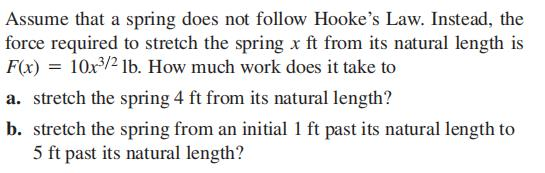### Still have math questions?

Trigonometry
QuestionAssume that a spring does not follow Hooke's Law. Instead, the force required to stretch the spring $$x ft$$ from its natural length is $$F ( x ) = 10 x ^ { 3 / 2 } lb$$ . How much work does it take to

a. stretch the spring $$4 ft$$ from its natural length?

b. stretch the spring from an initial $$1 ft$$ past its natural length to $$5 ft$$ past its natural length?

$$\text { (a) } 128 ft - lb \quad \text { (b) } 219.6 ft - lb$$